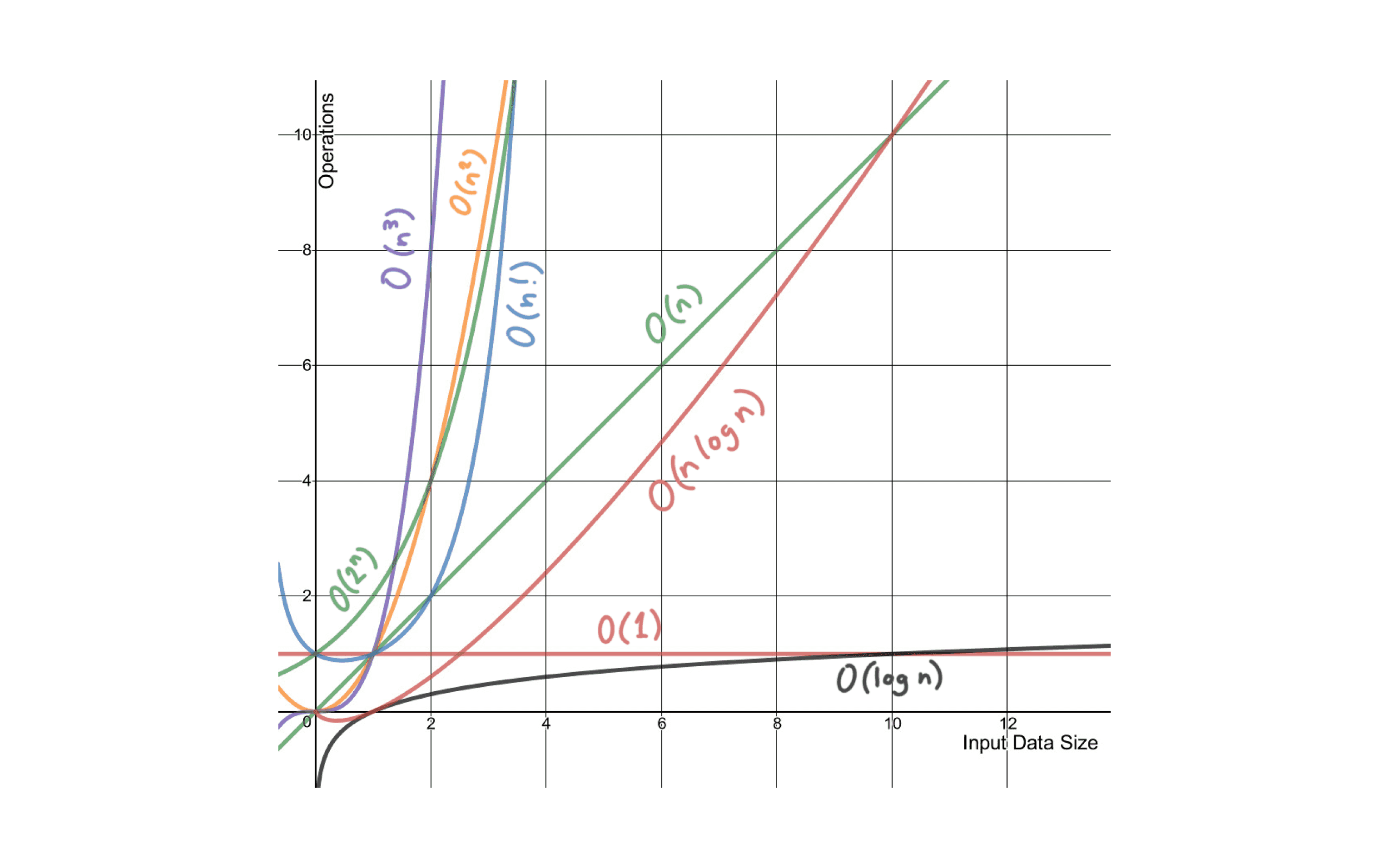We launched our first course, Introduction to Data Structures in C++! Learn more 🎉

Fundamentals

# What is time complexity?

June 29, 2022To become an efficient programmer, you must know how to evaluate the effectiveness of the program/algorithm. Using space and time complexity can help you do this and make your program behave in the required optimal conditions.

### What does Time Complexity mean?

Time complexity is the amount of time taken by an algorithm to run, as a function of the length of the input. It measures the time taken to execute each statement of code in an algorithm.

For an example, if I write this program:

int main() {

print("Hello"); //statement 1

}

It executes one statement so the time complexity of this program will be O(1).

### You must be wondering what is this O(1) right?

We cannot look at a C++ program and its input and say, “This task will take 3.21 seconds,” unless we know which machine and which compiler will be used. Moreover, even if we know the program, the input, the machine, and the compiler, it is usually far too complex a task to predict exactly the number of machine instructions that will be executed. For these reasons, we usually express the running time of a program using “big-oh” notation [O(n)], which is designed to let us hide constant factors such as:

1. The average number of machine instructions a particular compiler generates.

2. The average number of machine instructions a particular machine executes per second.

When comparing algorithm performance, we are interested in the number of operations that an algorithm performs. This is called time complexity.

In this model, we consider that each basic operation (addition, multiplication, comparison, assignment, etc.) takes a fixed amount of time, and we count the number of such operations.

We can usually express this number as a function of the size of the input, which we call n. And sadly, this number usually grows to infinity with n (if it doesn't, we say that the algorithm is O(1)). We separate our algorithms in big speed classes defined by Big-Oh : when we speak about a "O(n^2) algorithm", we mean that the number of operations it performs, expressed as a function of n, is a O(n^2). This says that our algorithm is approximately as fast as an algorithm that would do a number of operations equal to the square of the size of its input, or faster.### Best, Average, and Worst case complexities

Best, worst and average case is something we come across everyday. For every decision you implement, there's a best case, worst case and an average case.

For example, you want to run in a marathon. You will definitely finish the marathon, but how quickly and with how much effort? Well that would depend on a lot of factors. The best case for you would be if the track is short and smooth, you're familiar with it and you own Nike Air Jordans. The worst case would be if the path is made of fire and you have to walk through it barefoot. And an average situation would be if you get a regular track with decent running shoes.Similarly, for algorithms too, we have a best case, worst case and average case scenario.

Best-case time complexity

The best case in which an algorithm performs, for example if you are trying to find any number(k) in an array(arr), chances are you will find k in the first position itself.

Average-case time complexity

The average time an algorithm may take. It's often harder to compute, and it also requires knowledge of how the input is distributed. For the above example, if k does not lie at the end, you may say it will take some average time.

Worst-case time complexity

It is the time take by the algorithm, given the worst case. Like in the above example, if k is the last element of the array, you will say it will take worst time.

This is all about the basics of time complexity. The next question that would come to your mind is: How to calculate time complexity? Let's learn about it in the upcoming blogs!

Our first course, Introduction to Data Structures in C++ worth ₹499/month is available at a price of your choice for the first month access.

Offer available for a limited time

Subscribe

301/302, 3rd Floor, Saket Callipolis, Sarjapur Main Rd, Doddakannelli, Bengaluru, Karnataka 560035

© CodePark Pvt. Ltd. 2022

For any queries, reach out to us at hello@byteavenue.org

For any queries, reach out to us at hello@byteavenue.org

301/302, 3rd Floor, Saket Callipolis, Sarjapur Main Rd, Doddakannelli, Bengaluru, Karnataka 560035

© CodePark Pvt. Ltd. 2022

For any queries, reach out to us at hello@byteavenue.org

301/302, 3rd Floor, Saket Callipolis, Sarjapur Main Rd, Doddakannelli, Bengaluru, Karnataka 560035

© CodePark Pvt. Ltd. 2022